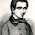## Wednesday, July 18, 2018

### Electric charge and local fields

Studying electric charge requires mathematical structures distinct from spacetime. Charge is conserved, but that conservation law is not related to any transformation of space or time. To measure electric fields, we need functions that measure something other than space and time.

The simplest type of such function would be from spacetime to the complex numbers. But such a function has an immediate problem with the locality axiom. A function like that would allow comparing values at spatially separated points, but no such comparison should be possible. It doesn't make sense to relate physics at one spacetime point to anything outside its light cones.

So we need to have a way of doing functions on spacetime, such that function values can only be compared infinitesimally, or along causal curves. The mathematical construction for this is called a line bundle.

For electromagnetism, imagine that every point in spacetime has a phase, a complex number with norm one. The phase does not mean anything by itself, but the change in phase along a causal curve is meaningful, and is the key to describing the propagation of an electromagnetic field.

A bundle connection is, by definition, the mathematical info for relating the phases along a curve. It is usually given in infinitesimal form, so the total phase change along the curve is given by integrating along the curve.

It turns out that a bundle can be curved, just as spacetime can be curved. The curvature is a direct measure of how the phase can change around a closed loop.

You cannot make a flat map of the surface of the Earth, without distorting distances, and you can make a flat map of a curved bundle either. You can choose some function (called a bundle section) that defines a preferred phase at each point. Then any other section is this one multiplied by some complex function on spacetime. Thus any section can be written fs, where f is an ordinary complex function on spacetime, and s is a special section that gives a phase at each point. You can think of the phase as a norm-1 complex value, but that is misleading because the values at one point cannot be directly compared to the values at other points.

For derivatives, we expect a product formula like d(fs) = f ds + s df.

The difficulty is in interpreting ds, as a derivative requires comparing values to nearby points. A bundle connection is what allows infinitesimal comparisons, so in place of ds there is some ω for which we have a formula: d(fs) = f ω + s df.

The ω is what mathematicians call a connection 1-form, and what physicists call a 4-vector potential. It is not necessarily the derivative of a function. The derivative is what mathematicians call the curvature 2-form, and what physicists call the electromagnetic field. It does not depend on the choice of the section s.

Maxwell’s equations reduce to interpreting the derivative of the curvature as the charge/current density.

The whole theory of electromagnetism is thus the inevitable consequence of looking for a geometric theory obeying locality.

One can also construct field theories with higher dimensional bundles, replacing the complex numbers C with Cn for some n, and the phase with U(n), the group of nxn unitary matrices. Other groups are possible also, such as SU(n), the group of such matrices with determinant 1. The 1-form ω has values in the Lie algebra, which is the skew symmetric complex matrices, if the group is U(n).

When a field theory is quantized, notorious infinities arise. For field theories based on connections on bundles as above, there is a theory of renormalization to cancel the infinities. It is a generalization of the system for quantum electrodynamics. Other theories are difficult or impossible to renormalize. Physicists settled on gauge theories because they are the only ones to give finite predictions in a quantum theory.

Historical note. This formulation of Maxwell’s equations appears to have been known by Hermann Weyl and other by 1920 or so, but it may not have been explicitly published until 1970 or so.

1.Charge is not math. Nor is the charge field. Until you have an underlying theory that can explain what these things are besides '+' and '-' signs in a diagram, then you are diddling with epicycles. This is why physics is so screwed up, too much unassigned math.

1.Mathematicians are constantly muddying the waters with ugliness and ad hoc amateur shows. In clifford algebra, multiplication by i is just a rotation by 90 degrees, and multiplying twice by i is a rotation by 180 degrees (negation). Not to get into the topic of normed division algebras but mathematicians just invent junk they don't even understand. They make a mess and refuse to clean it up.
https://slehar.wordpress.com/2014/03/18/clifford-algebra-a-visual-introduction/
https://slehar.wordpress.com/2014/06/26/geometric-algebra-projective-geometry/
https://slehar.wordpress.com/2014/07/24/geometric-algebra-conformal-geometry/

2."The whole theory of electromagnetism is thus the inevitable consequence of looking for a geometric theory obeying locality."

It is well established that photons of light are electromagnetic fields.

Are you saying that photons thus obey locality, and can never be entangled?

3.Dear Roger,

``When a field theory is quantized, notorious infinities arise.''

Must be so only in the relativistic settings, right? Can you provide a simpler example, or even a made-up (hypothetical case) example, but in the non-relativistic settings?

In particular, does the idea of the self-force on a point-particle electron illustrate the cancelling of infinities you are talking about?

Do you have any further explanation or comment to offer on the second answer (the one by ``mcFreid'') here: https://physics.stackexchange.com/questions/99285/the-problem-of-self-force-on-point-charges . Thanks in advance.

Best,

--Ajit